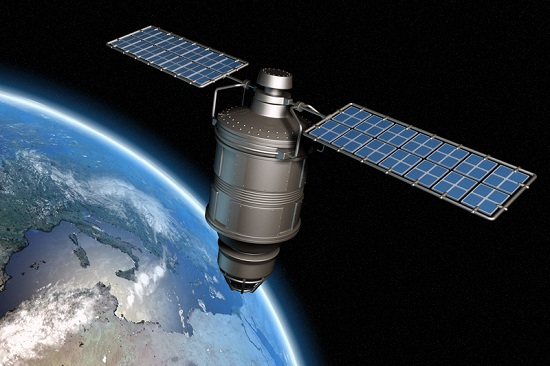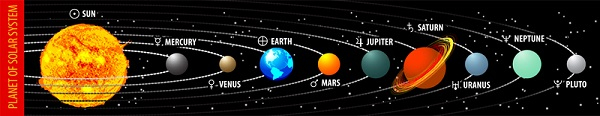# Energy Orbiting Satellite

## Introduction

All the planets in the solar system are found at different distances. The first four planets are closer to each other and closer to the Sun. They form the inner solar system. The planets in the outer solar system are far away from the Sun. Remote censoring is the collection of information about objects on Earth from a distance without direct contact. It has a long history, beginning in the 28th and 19th centuries with photographic instruments mounted on balloons and pigeons’ legs and continuing through the era of remote sensing. During the 20th century, aerial photography and satellite remote sensing technology developed rapidly.

## What is Satellite?

An object that orbits the planets in the solar system is called a satellite. We call natural satellites moons to distinguish them from man-made satellites. We can see it because the light that falls on our Earth’s natural satellite, the Moon, is reflected. Due to gravity, these orbits the planets. All the planets in the solar system have moons except Mercury and Venus. We have previously learned about the natural satellites orbiting the planets. Gravitational force acts between the planet and the satellite.

Nowadays many satellites are placed in orbit around the earth. Sputnik launched in 1956 was the first artificial satellite to be launched. Satellites are launched to orbit the Earth at an altitude of a few hundred kilometres.

After taking the satellite to such an altitude that wind friction is negligible. After being taken to this altitude, if the satellite is given a horizontal velocity it will move in a near circuit orbit.Fig. 1. Satellite

## Satellite Communication

Signal transmission between transmitter and receiver is the type of communication used in satellite communication. The information signal is transmitted from the earth station to a satellite in the sky over an uplink (frequency band). It is then retransmitted to another earth station through the downlink (frequency band) amplified by a transponder there.

## Types of Satellites

Satellites are divided into various categories based on their applications. Some of the satellites are described below

### Weather Satellites

These satellites are used to monitor the Earth’s weather and climate. These satellites help us predict rainfall, dangerous cyclones, and storms by measuring the mass of clouds.

### Communication Satellites

Television, radio, and internet signals are transmitted by these satellites. For longdistance transmission, more than one satellite is used.

Navigation Satellites are involved in locating the geographical location of ships, aircraft, or any other object.

## What are Orbit and their types?

Planets revolve around the Sun in a fixed curved path. These paths are called orbits. Orbit is the path around a centred particle or system due to the gravitational pull of other particles or objects.Fig.2. Orbit of the planet

There are three orbits around Earth. They are

• LEO - Low Earth orbit

• MEO - Medium Earth orbit

• High Earth orbit

They are determined by the altitude of the earth's orbit. The orbit with an altitude up to 2000km or 0-1240 miles is called Low earth orbit. Intermediate orbit is the other name for MEO. It is in the middle of LEO and geosynchronous orbit.

The altitude range of MEO is up to 2000km to 35,786 kilometers. 12 hours is the orbital period of Medium Earth Orbit. The orbits which are ranging more than geosynchronous orbit are called High Earth orbits. The altitude range of the High Earth Orbit is 35,786 km.

## What is the Energy of Orbiting Satellites?

The energy of orbiting satellites is defined as the energy needed to revolve around the earth. It has both kinetic energy and potential energy because it revolves around the earth and it is in the gravitational field of the Earth.

$$\mathrm{Energy\:=\:Kinetic\:energy\:+\:Potential\:Energy}$$

## Energy Relationship for Satellites?

The total energy of a satellite orbiting the Earth at a height ‘h’ above the Earth’s surface is calculated as follows.

The Potential energy of a satellite,

$$\mathrm{U=-\frac{GM_{s}M_{E}}{(R_{E}+h)}\:\:------(1)}$$

Where,

$$\mathrm{M_{s}\:-Mass\:of\:the\:satellite}$$

$$\mathrm{M_{E}\:-Mass\:of\:the\:Earth}$$

$$\mathrm{R_{E}\:-Radius\:of\:the\:satellite}$$

The Kinetic energy of the satellite

$$\mathrm{K.E=\frac{1}{2}M_{s}V^{2}\:\:------(2)}$$

Here ‘v’ is the orbital velocity of the satellite. The orbital velocity of a satellite depends on its height above the Earth. The closer it is to the Earth, the faster it must be moving. A satellite at a distance of 200 km would have to travel at a speed of slightly more than 27,400 km/h. While operating like this it will circle the earth in 24 hours.

The satellite appears to be in the same place above the Earth’s surface. Thus, since they are in the same position concerning the Earth, these types of satellites are called geostationary satellites. The orbital velocity (v) is given as,

$$\mathrm{V=\sqrt{\frac{GM_{E}}{(R_{E}+h)}}}$$

$$\mathrm{G\:-\:Gravitational\:Constant}$$

$$\mathrm{M_{E}\:-\:Mass\:of\:the\:Earth}$$

$$\mathrm{R_{E}\:-\:Radius\:of\:the\:Earth}$$

$$\mathrm{h\:-\:Height\:of\:the\:satellite\:above\:Earth's\:surface}$$

Substitute the value of ‘v’ in equation (2)

$$\mathrm{K.E=\frac{1}{2}\frac{GM_{S}M_{E}}{(R_{E}+h)}}$$

Therefore total energy of the Satellite

$$\mathrm{E=\frac{1}{2}\frac{GM_{S}M_{E}}{(R_{E}+h)}-\frac{GM_{S}M_{E}}{(R_{E}+h)}}$$

$$\mathrm{E=-\frac{GM_{S}M_{E}}{2(R_{E}+h)}}$$

Here the negative sign indicates that the satellite is covered by the earth’s gravitational field. Therefore the satellite cannot get out of the Earth’s gravitational field. The total energy of the satellite is zero when ‘h’ reaches infinity. This means that the satellite is completely free from the dominance of the Earth’s gravitational field. And at a great distance means the satellite is not bound to Earth.

## The Orbital Period of Satellites

The orbit period of a satellite is defined as the time taken by a satellite to complete one orbit around the earth.

$$\mathrm{Orbital\:period\:T\:=\:\frac{Distance\:travelled}{Orbital\:velocity}}$$

$$\mathrm{T=\frac{2\pi r}{v}}$$

Substituting the value of ‘v’

$$\mathrm{T=\frac{2\pi r(R+h)}{\sqrt{\frac{GM_{E}}{(R_{E}+h)}}}}$$

Simplify the above equation by Kepler’s law, the orbital period of the satellite

$$\mathrm{T=2\pi \sqrt{\frac{R_{E}}{g}}}$$

Substituting the values of R and g, the orbital period of satellite T$\mathrm{\cong }$85 minutes.

## Conclusion

Remote censoring is the collection of information about objects on Earth from a distance without direct contact. An object that orbits the planets in the solar system is called a satellite. We call natural satellites moons to distinguish them from man-made satellites. Signal transmission between transmitter and receiver is the type of communication used in satellite communication. Weather satellites, communication satellites, and Navigation satellites are types of satellites based on their applications.

## FAQs

Q1. Why are satellites also called radio repeaters?

Ans. Communications satellites help rebroadcast radio signals through transponder downlinks to reach distant locations. Hence it is also called a radio repeater in the sky. It has applications in all fields.

Q2. What is GPS?

Ans. GPS stands for Global Positioning System. GPS has become so indispensable in our vehicles and mobile phones that we feel like we are missing something. It is the world’s first and currently the most widely used GNSS. It was developed by the United States Department of Defense.

Q3. What is an international space station?

Ans. The international space station is a large space station that houses astronauts. It operates in a low Earth orbit at a distance of about 400 kilometers. It also serves as a science laboratory.

Q4. What are the uses of robotic arms?

Ans. Robotic arms support research at the international space station, by removing tumors that cannot be surgically removed (eg. Brain tumors) and performing biopsies with high precision. Its creators claim that such tissue studies can be performed.

Q5. What are asteroids?

Ans. There is a large gap between the orbit of Mars and the orbit of Jupiter. In this interstellar space, millions of pieces of rock (which appear like a band) are orbiting around the planets formed when they formed. These are called asteroids.

Updated on: 17-Jan-2023

212 Views